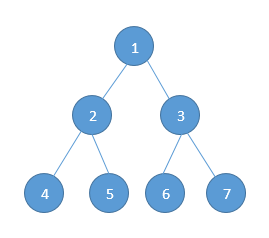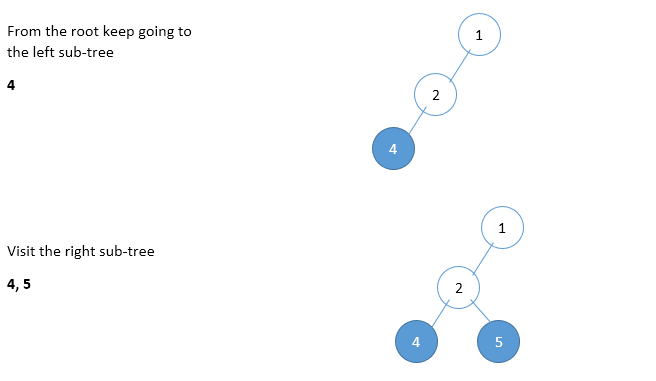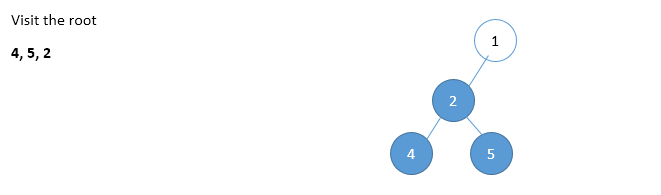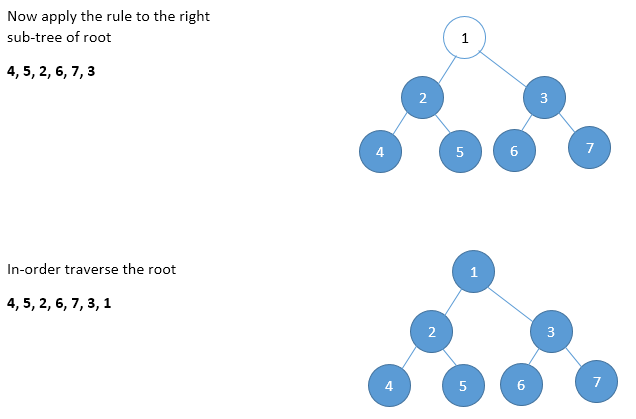Home Theory PostOrder Traversal in a binary tree

# PostOrder Traversal in a binary tree

0 comment

We discussed about the basic tree traversals in this post –

Lets take our own sample tree for understanding post-order traversal.In Post-Order traversal, the root is visited after both sub-trees are completed. Post-order traversal is defined as follows:-

• Traverse the left sub-tree in post-order. (Step 1)
• Traverse the right sub-tree in post-order. (Step 2)
• Visit the root

The post-order traversal can then be defined in this way –The nodes of the tree will therefore be in order – 4, 5, 2, 6, 7, 3, 1

The recursive version can be written as:-

void postOrderRecursive(struct binaryTreeNode * root)
{
if(root)
{
// Post-order traversal of left sub-tree (Step 1)
postOrderRecursive(root -> left);

// Post-order traversal of right sub-tree (Step 2)
inOrderRecursive(root -> right);

// Visit the root (Step 3)
printf("%d ", root -> data);
}
}


### Non-recursive Post-order traversal

In pre-order and in-order traversals, after popping the stack element we do not need to visit the same vertex again. But in post-order traversal, each node is visited twice. That means, after processing left sub-tree we will be visiting the current node and also after processing the right sub-tree we will be visiting the same current node. But we should be processing the node during the second visit. Here the problem is how to differentiate whether we are returning from left sub-tree or right sub-tree ?

Trick for this problem is: after popping an element from the stack, check whether that element and right of top of the stack are same or not. If they are same then we are done with processing of left sub-tree and right sub-tree. In this case we just need top pop the stack one more time and print its data.

void postOrderNonRecursive(struct binaryTreeNode * root)
{
// Create a new empty stack
struct stackNode * S = NULL;

// We need to run the loop until stack is not empty again
while(1)
{
if(root != NULL)
{
// If a node exists, we add it to the stack
S = push(S, root);
root = root -> left; // Step 1
}
else
{
if(isStackEmpty(S))
{
return;
}
else
{
if(S -> top -> right == NULL)
{
root = S -> top;

// Pop the stack
S = S -> next;

printf("%d ",root -> data); // Step 3

if(root == S -> top -> right)
{
printf("%d ",S -> top -> data);
S = S -> next;
}
}
}
if(!isStackEmpty(S))
root = S -> top -> right; // Step 2
else
root = NULL;
}
}

// We need to free the stack memory
free(S);
}


Time Complexity:- O(n)
Space Complexity:- O(n)

#### You may also like

This website uses cookies to improve your experience. We'll assume you're ok with this, but you can opt-out if you wish. Accept Read More